# Use OFFSET and COUNTA to create dynamic ranges with automatically updating formulas in Excel 2007 and Excel 2010

Robbie mostly writes about Skyrim but also occasionally sheds light on the oddities of Microsoft applications such as Excel and Outlook.

In this article, we are going to look at something that is rather cool and something I have needed in my own Excel Spreadsheets for some time.

Imagine if you will, a spreadsheet where you record invoices, sales, web hits, etc, and then SUM them to give yourself a running total. Every time you add extra rows to your spreadsheet you have to update your SUM formula to allow for the extra items.

Well not anymore! Welcome to the dynamic range using the OFFSET and COUNTA functions. Using this function, whenever rows are added (or deleted) and also each time copied cells are inserted, the SUM formula will automatically and dynamically update to reflect this.

## Using the OFFSET Function in a Formula in Excel 2007 and Excel 2010

The OFFSET function allows you to ask Excel to tell you the contents of a cell (or a range of cells) a certain number of rows and columns away from a reference cell (or cells).

If you use OFFSET on its own, it will return the value of the cell you referred it to. We will start with that as an example:

=OFFSET(B3,1,1,1,1)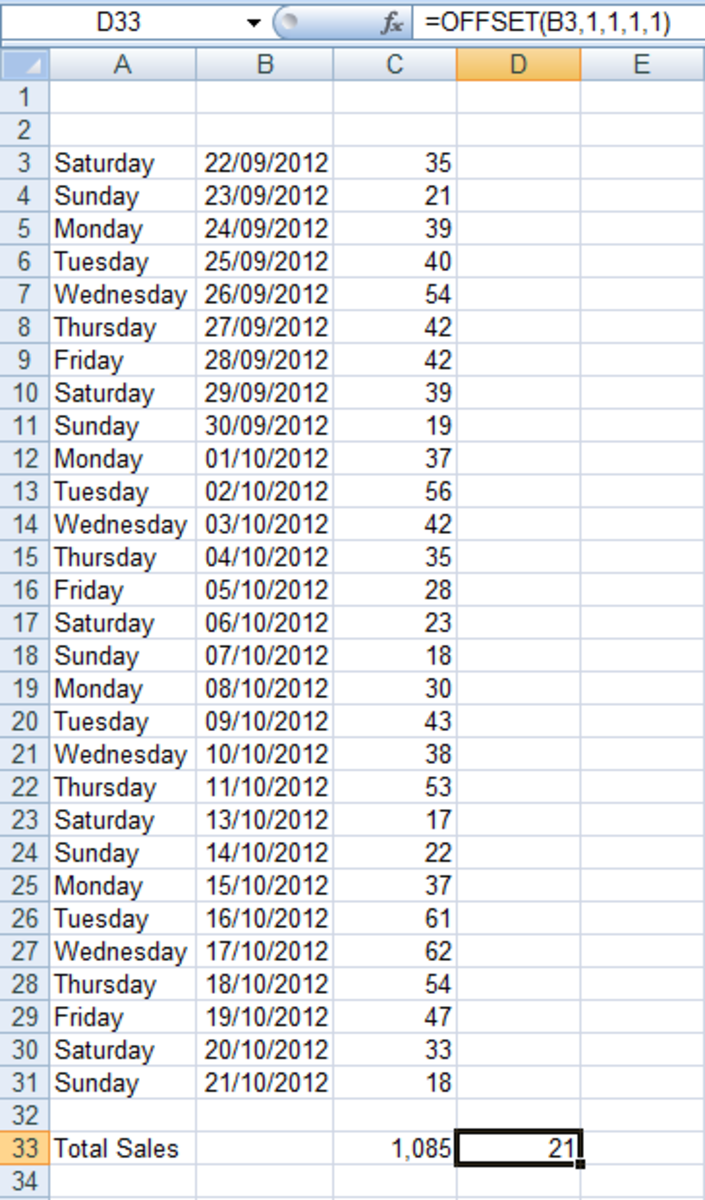Example of how to use the OFFSET function in a formula in Excel 2007 and Excel 2010.http://robbiecwilson.hubpages.com/

The formula is made up of the following parts:

• The cell you are asking Excel to start from (in this instance B3) (known by Excel as the Reference)
• The next part of the formula is the number of Rows (which can be either up or down) from the reference cell to the cell you are looking at. We are interested in cell C4 so this value is 1 as it is one row down from cell B3.
• Next, we need to know how many Columns from the starting to the reference cell. This is 1 again as it is one column from B3 to C4
• The fourth element of the formula is Height. This is the number of rows you want the returned data to be.
• Finally, we have Width or the number of columns you want the data to be.

Note: Rows and Columns can be a negative or positive number. Please refer to the below table to understand when to use positive or negative values.

## When to Use Positive and Negative Numbers for Rows and Columns in OFFSET formulas

 Row Column Positive Below Reference Right of Reference Negative Above Reference Left of Reference

Note: Height and Width must both be positive. If they are not specified, Excel will use the height and width of the reference.

Note: If you get a #VALUE you have typically asked Excel to display something it cannot display.

To illustrate this:

If I use the following formula:

Scroll to Continue

=OFFSET(B3,1,1,2,1)

I am asking Excel to display the results of one cell in two cells which it cannot do so it returns an error.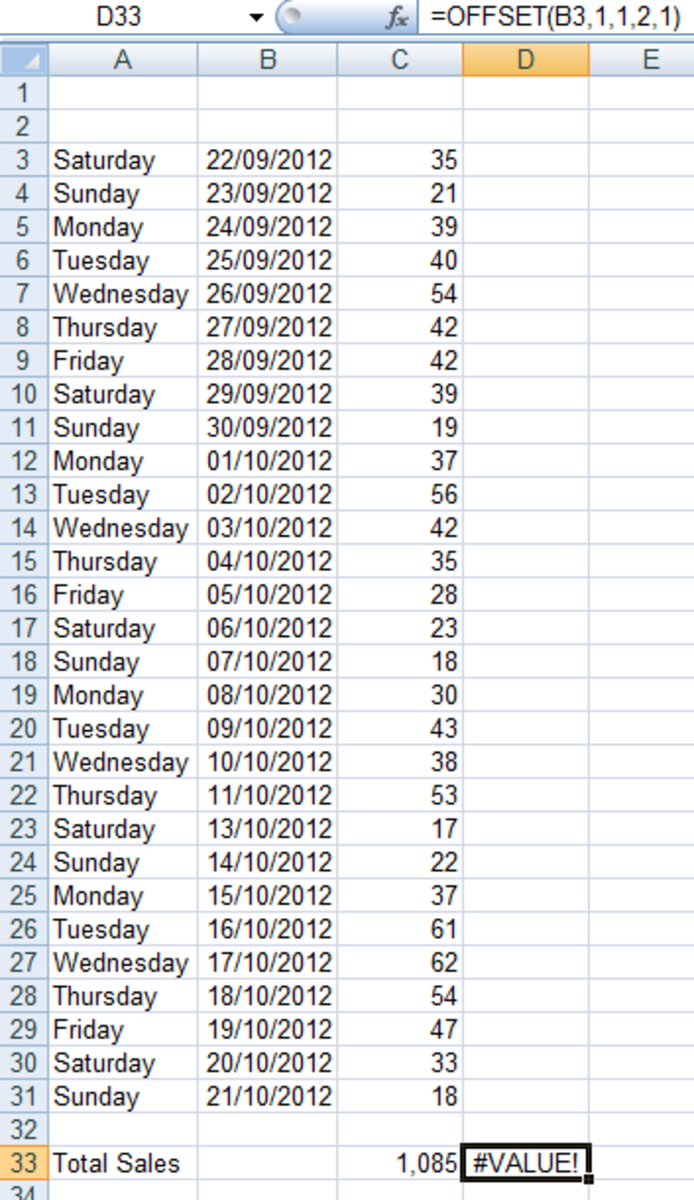Example of a #VALUE error received when using the OFFSET function in a formula in Excel 2007 and Excel 2010.http://robbiecwilson.hubpages.com/

Note: If you get a #REF error you have gone off the edge of your worksheet.

This formula results in a #REF error, as there are no such row four rows above B3.

=OFFSET(B3,-4,2,1,1)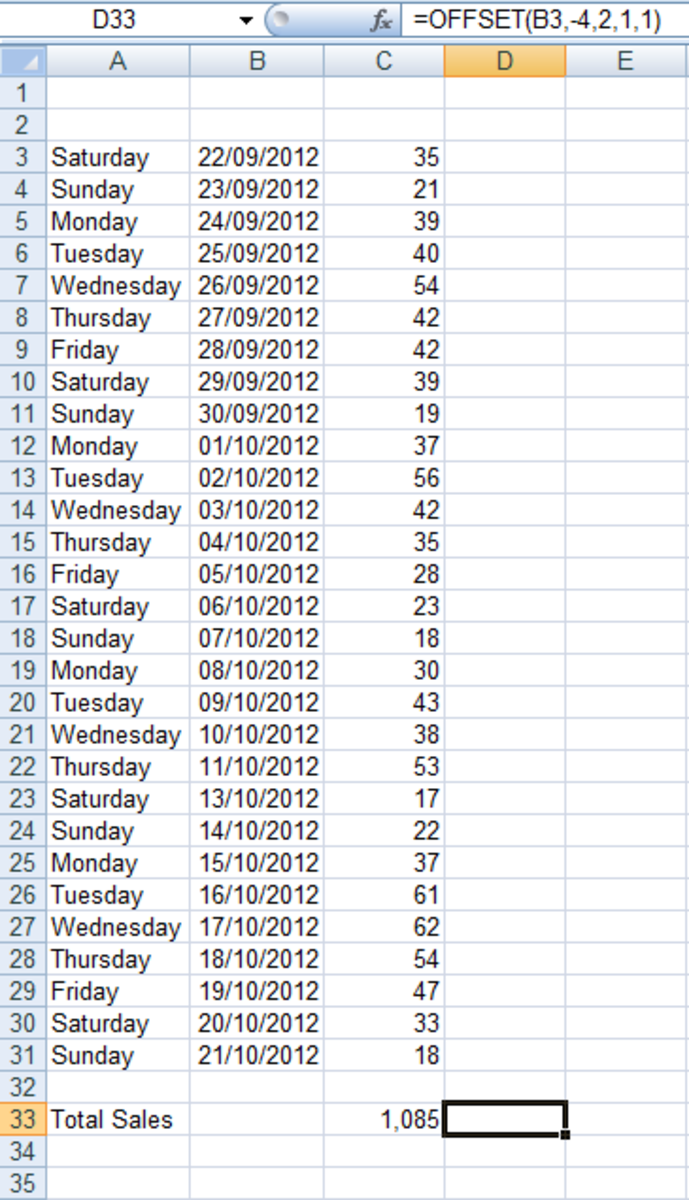Example of a #REF error received when using the OFFSET function in a formula in Excel 2007 and Excel 2010.http://robbiecwilson.hubpages.com/

## Using OFFSET With COUNTA in a Formula to Create a Dynamic Range in Excel 2007 and Excel 2010

Now that we understand the OFFSET function and how to use it in a formula, we get to the really interesting part of this article; we get to make a dynamic range using it alongside COUNTA. We can then SUM the values in the dynamic range and this formula will automatically recalculate if cells are added, removed or their contents changed. Of course, SUM is just an example that I chose for this article, you could use AVERAGE, MAX or any other function you felt was appropriate for your needs alongside the OFFSET and COUNTA functions.

First, let’s quickly look at what COUNTA does for us in this example:

It simply counts the number of cells that are not blank between the start and end cell (in this case there are 29 non blank cells between cell C3 and cell C32).

=COUNTA(C3:C32)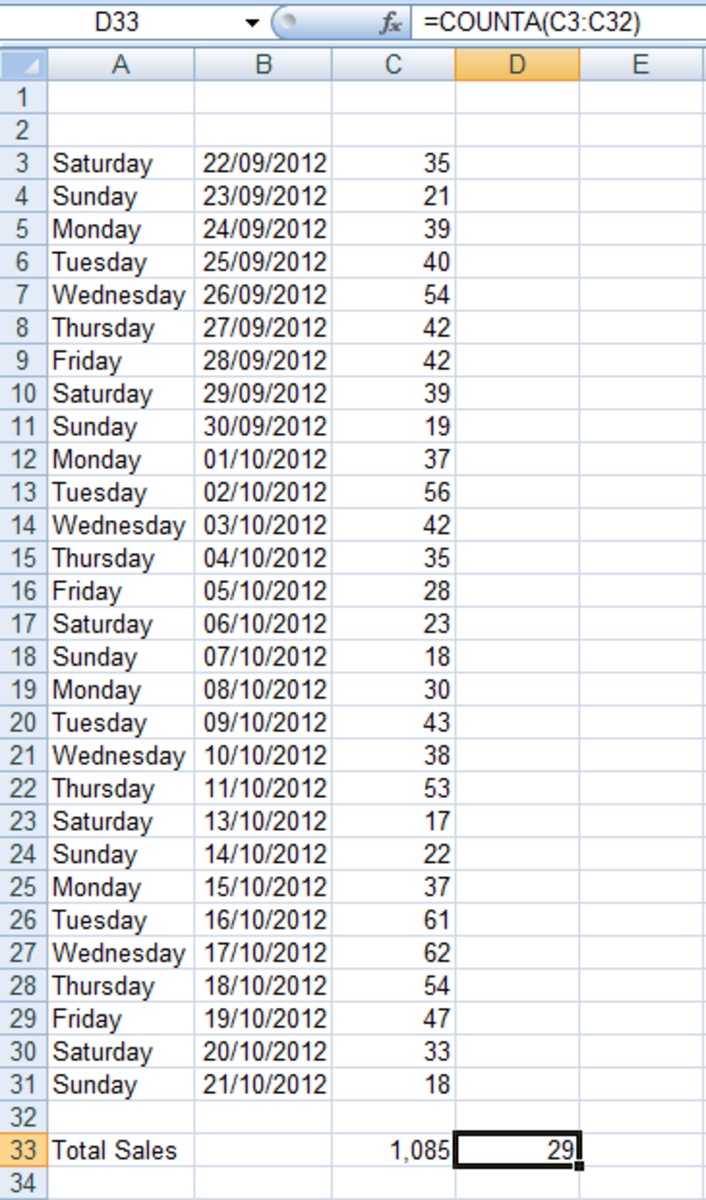Example of the COUNTA function used in a formula in Excel 2007 and Excel 2010.http://robbiecwilson.hubpages.com/

Now, we include the COUNTA formula above as part of our OFFSET formula to create a dynamic range. The finished formula is:

=SUM(OFFSET(C3,0,0,COUNTA(C3:C32),1))

We will begin by looking at the OFFSET section of the formula:

OFFSET(C3,0,0,COUNTA(C3:C32),1)

• We start at cell C3 as our Reference
• The number of Rows we are asking Excel to move from the starting cell of C3 is 0
• Likewise, the number of Columns is also 0 (I like to add and remove columns to my data so keeping it at 0 means that the formula will never break)
• Now comes the dynamic part of the whole formula. For the Height, we are telling Excel that the height is the result of the COUNTA formula which is currently 29. Should we add or remove rows, this will adjust automatically!
• Finally, we advise Excel of the Width, which is in this case 1 column.

Now that we understand the OFFSET section of the formula we add the SUM function to it to complete the overall formula giving us:

=SUM(OFFSET(C3,0,0,COUNTA(C3:C32),1))

If we now Insert an additional blank row you will notice that the Total Sales goes down by 18 (which is the value in cell C32 which is the final row) from 1,085 in the figure above to 1,067 in the figure below.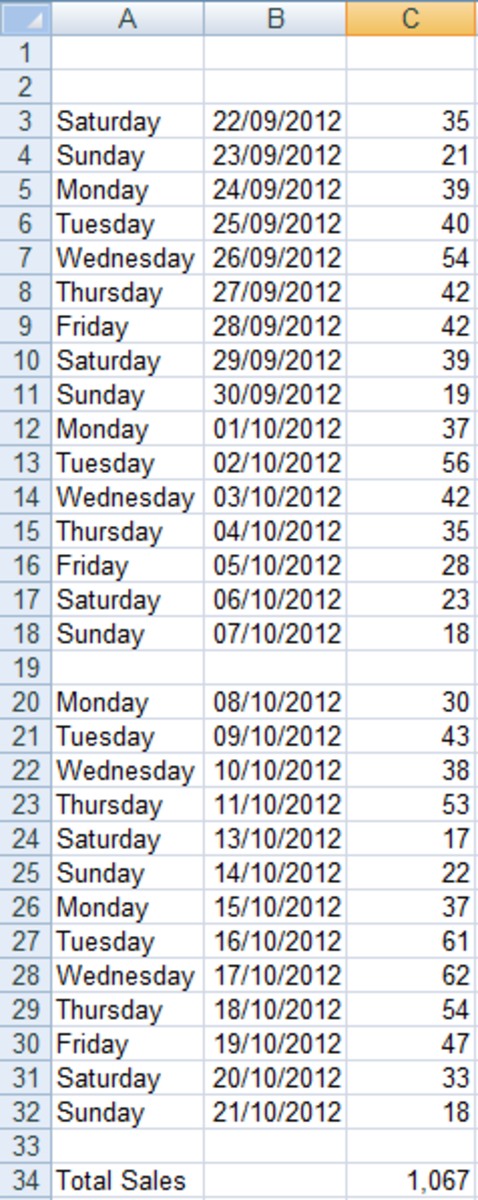Inserting a blank row into a dynamic range will lead to formulas being inaccurate in Excel 2007 and Excel 2010.http://robbiecwilson.hubpages.com/

I now add a value into the blank row and the Total Sales is now correct and includes the value in C32.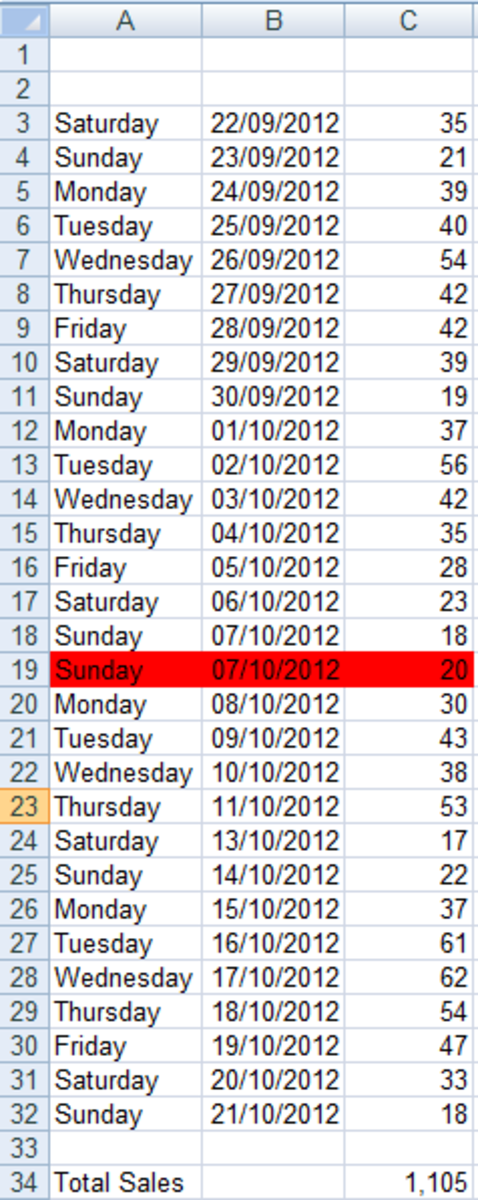With data entered into cell C19, the formula calculating the values in the dynamic range in Excel 2007 and Excel 2010 is now correct.http://robbiecwilson.hubpages.com/

Note: Inserting a blank row into your dynamic range will lead to the results shown in your SUM formula being incorrect.

Note: Should you add additional rows, for example by Inserting Copied Cells Excel will simply increase the COUNTA part of the formula which makes it dynamic.

By way of an example, I added four rows to my spreadsheet and Excel updated my formula automatically!

=SUM(OFFSET(C3,0,0,COUNTA(C3:C36),1))

## Dynamic Ranges in Excel

The ability to have dynamic ranges in your Excel spreadsheets containing data such as sales, invoices, web hits etc which can then be added up or averaged is a very useful thing indeed. This means that you no longer have to manually update your formulas whenever you make additions or deletions to your data.

To achieve this:

• We started by using the OFFSET function in the formula. This function allows you to start with a reference cell (or cells) and ask Excel to display the contents of another area a specific number of rows and columns from the reference cell
• Next, we added the COUNTA function to provide the Height of the area for OFFSET. Whenever cells are added or removed from the range, the COUNTA section of the formula is updated automatically.
• By adding the SUM function to this formula, we can now add up the cells that Excel has displayed via OFFSET.

When all of these functions are added to a formula, it will now dynamically sum a range of cells, updating automatically when data is added or deleted from the range. This for me is the beauty and power of Excel, the fact that you can add two functions together and create something that is not only very useful, but also more than the sum of its parts.

I hope that you have found this article useful and informative and that using OFFSET will transform your spreadsheets in the same way that it has transformed mine. Many thanks for reading, please feel free to leave any comments you may have below.